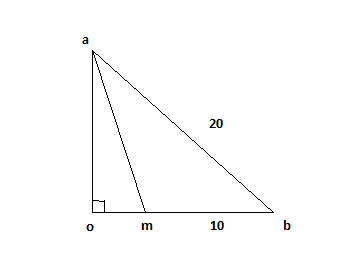Question 33

# Euclid has a triangle in mind. Its longest side has length 20 and another of its sides has length 10. Its area is 80. What is the exact length of its third side?

SolutionWe know that area = 0.5*h*10 ; we get h = 16=oa.

Using pythagoras we find

$$ob^2+oa^2 = ab^2$$

$$ob^2+16^2 = 20^2$$

ob = 12

Using pythagoras in triangle oam we get

am = $$\sqrt {2^2 + 16^2 }$$ = $$\sqrt {260}$$.